# Case Study of MINT sPLS-DA with Stem Cell dataset

The aim of this P-integrative analysis is to generate a model which can classify novel samples into one of a set of stem cell classes based on their genomic information.

# Rscript

The R script used for all the analysis in this case study is available here.

# To begin

Load the latest version of mixOmics.

library(mixOmics) # import the mixOmics library


## The data

In a previous study, 15 transcriptomics microarray stem cells datasets were assessed to classify three types of human cells: human Fibroblasts (Fib), human Embryonic Stem Cells (hESC) and human induced Pluripotent Stem Cells (hiPSC). This case study operates on a subset of the available data, which was fully explored and analysed in . Only 4 of these 15 studies are included (125 samples).

There exists a biological hierarchy among the three cell types. On the one hand, differences between pluripotent (hiPSC and hESC) and non-pluripotent cells (Fib) are well-characterised and are expected to contribute to the main biological variation. On the other hand, hiPSC are genetically reprogrammed to behave like hESC and both cell types are commonly assumed to be alike. This MINT analysis addresses both subclassification problems in a single analysis.

The mixOmics stem cells dataset is accessed via stemcells and contains the following:

• stemcells$gene (continuous matrix): 125 rows and 400 columns. The expression levels of 400 different gene loci for 125 samples. • stemcells$celltype (categorical vector): length of 125. Indicates the cell type of each sample. Includes Fibroblast, hESC and hiPSC.
• stemcells$study (categorical vector): length of 125. Indicates which of the 4 studies the sample is drawn from (using an integer between 1-4). To confirm the correct dataframes were extracted, the dimensions are checked. The distribution of class labels is also examined. It can be seen that these class labels are not balanced across differing studies. data(stemcells) # extract stem cells data X <- stemcells$gene # use genetic expression levels as predictors
Y <- stemcells$celltype # use stem cell type as response study <- stemcells$study # extract study allocation of samples

dim(X)

##  125 400

summary(Y)

## Fibroblast       hESC      hiPSC
##         30         37         58

table(Y,study)

##             study
## Y             1  2  3  4
##   Fibroblast  6 18  3  3
##   hESC       20  3  8  6
##   hiPSC      12 30 10  6


# Initial Analysis

To start analysis, a basic MINT PLS-DA model will be formed, in which all features will be used to construct an arbitrarily chosen number of components (ncomp = 2).

# generate basic MINT pls-da model
basic.plsda.model <- mint.plsda(X, Y, study = study, ncomp = 2)

# plot the samples
plotIndiv(basic.plsda.model, legend = TRUE,
title = ' ', subtitle = ' ', ellipse = TRUE)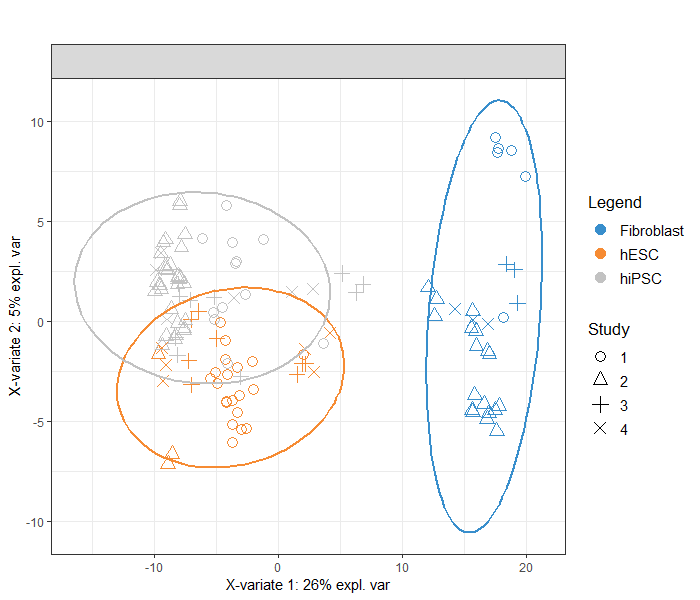FIGURE 1: Sample plot from the MINT PLS-DA performed on the stemcells gene expression data. Samples are projected into the space spanned by the first two components. Samples are coloured by their cell types and symbols indicate the study membership.

The first component is able to separate the Fibroblast cells from the other two groups quite well (Figure 1). Likely due to the similarity of hESC and hiPSC cells described above, these two groups are not well separated and have significant overlap, though the second component does a better job than the first in this regard. The presence of all 400 genetic features may be responsible for this.

This preliminary analysis is important as it informs the future models that will be generated, such that discrimination between these two latter groups is of greater weight than the fibroblast group.

## Basic sPLS-DA Model

Due to the lack of discriminative ability the MINT PLS-DA model has on this dataset, a MINT sPLS-DA will be used instead. The first step is to generate a basic model which includes a greater number of variables and components than necessary, such that these can be tuned. All features are included to begin (no keepX parameter passed) with and a total of five components (ncomp = 5) will be constructed.

basic.splsda.model <- mint.plsda(X, Y, study = study, ncomp = 5)


## Tuning the number of components

First, the optimal number of components to construct will be calculated. The perf() function is used to estimate the performance of the model using LOGOCV ('Leave One Group Out Cross Validation). Figure 2 shows the visualisation of this tuning

set.seed(5249) # For reproducible results here, remove for your own analyses

splsda.perf <- perf(basic.splsda.model) # undergo performance optimisation
plot(splsda.perf)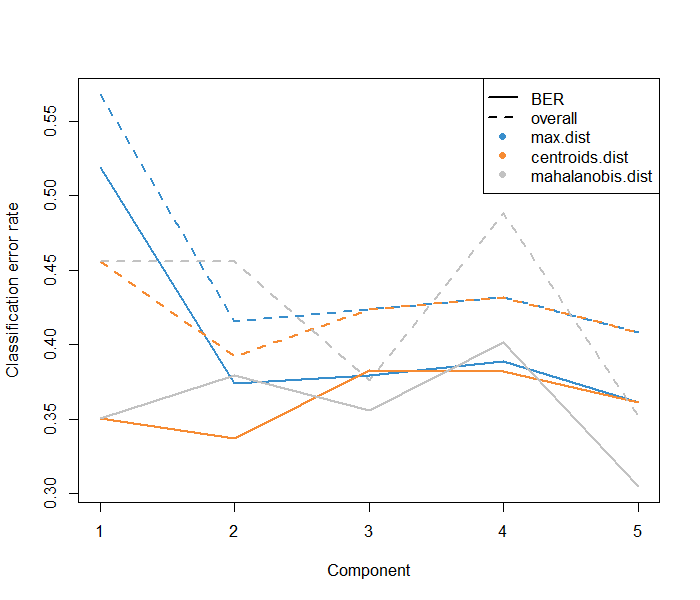FIGURE 2: Choosing the number of components in mint.splsda using perf() with LOGOCV in the stemcells study. Classification error rates are represented on the y-axis with respect to the number of components on the x-axis for each prediction distance

Below the optimal number of components to use is shown for each distance metric (both overall and balanced error rate). While all state that one component is best, purely for visualisation in this case study, two components will be used.

splsda.perf$choice.ncomp  ## max.dist centroids.dist mahalanobis.dist ## overall 1 1 1 ## BER 1 1 1  optimal.ncomp <- 2 # note that usually a call in the below line would be more appropriate: # optimal.ncomp <- splsda.perf$choice.ncomp["overall", "centroids.dist"]


## Tuning the number of features

To tune the number of utilised features, the tune() function is used. Again, this uses LOGOCV across the grid of inputted test.keepX values. Based on the mean classification error rate (overall error rate or BER) and a centroids distance, the optimal number of variables keepX to be included in the final model can be extracted. Below the optimal number of features to retain is printed.

splsda.tune <- tune(X = X, Y = Y, study = study,  # tune the number of features
ncomp = optimal.ncomp,# using optimal comp number
test.keepX = seq(1, 100, 1),
method = 'mint.splsda',
measure = 'BER', # balanced error rate
dist = "centroids.dist")

plot(splsda.tune, sd = FALSE)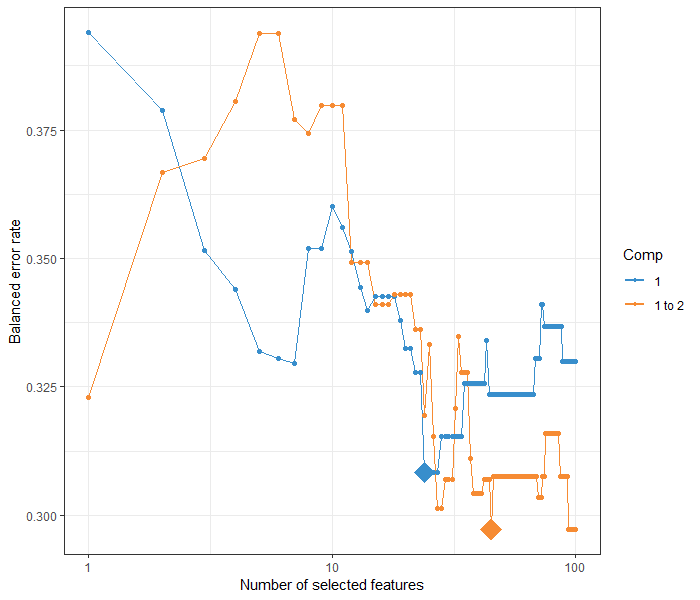FIGURE 3: Tuning plot of the MINT sPLS-DA models with up to 5 components. Diamonds represent the optimal number of features on a given component. Balanced error rate found on the vertical axis and is the metric to be minimised.

optimal.keepX <- splsda.tune$choice.keepX # extract optimal values optimal.keepX  ## comp1 comp2 ## 24 45  # Final Model Based on the tuning of component and feature count, a final MINT sPLS-DA model can be produced using the below call. Note once again, that in reality based off the results seen, ncomp = 1 is more appropriate. For this case study specifically, ncomp #generate optimal model using tuned parameters final.splsda.model <- mint.splsda(X = X, Y = Y, study = study, ncomp = optimal.ncomp, keepX = optimal.keepX)  # Plots ## Sample Plots The sample plot with the plotIndiv() function projects each sample into the space spanned by the components. This plot can depict all samples on the global components (Figure 4a) or each sample on the partial components associated with the study it was drawn from (Figure 4b). The visualisation of the partial components enables the examination of each study individually and check that the model is able to extract a good agreement between studies. Similar to the basic MINT PLS-DA model (Figure 1), the first component discriminates the Fibroblast group well while the second discriminates the hESC and hiPSC groups further, but not such that their 95% confident ellipses are non-overlapping (Figure 4a). The homogeny of clustering of each group across the four studies can be seen in Figure 4b. The second partial component of the study 2 and 4 seemed separated the hESC and hiPSC better than the in study 1 and 3. plotIndiv(final.splsda.model, study = 'global', legend = TRUE, title = '(a) Stem cells, MINT sPLS-DA', subtitle = 'Global', ellipse = T) plotIndiv(final.splsda.model, study = 'all.partial', legend = TRUE, title = '(b) Stem cells, MINT sPLS-DA', subtitle = paste("Study",1:4))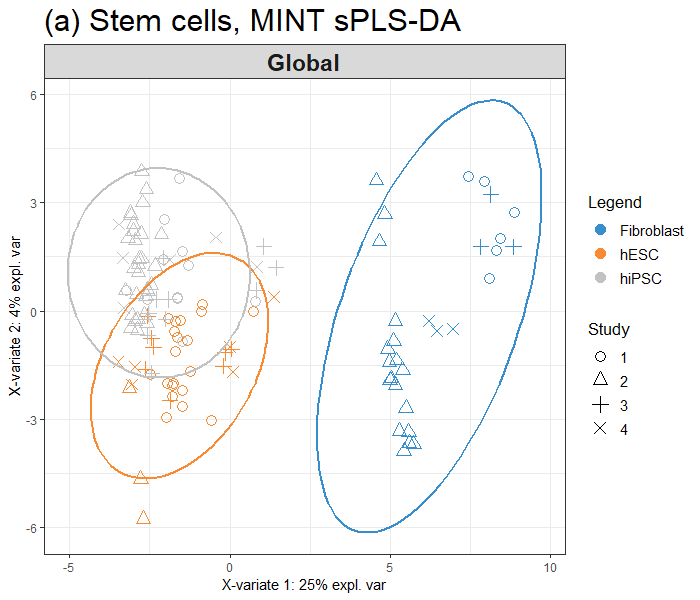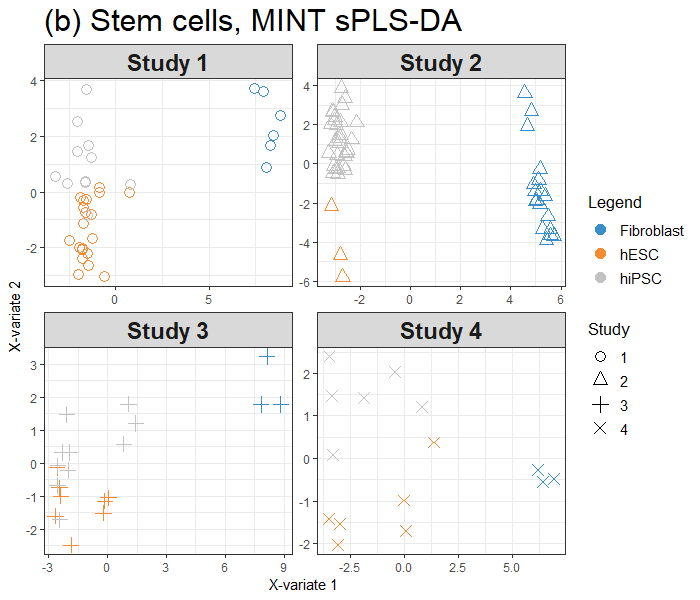FIGURE 4: Sample plots from the MINT sPLS-DA performed on the stemcells gene expression data. Samples are projected into the space spanned by the first two components. Samples are coloured by their cell types and symbols indicate study membership. (a) Global components from the model with 95% ellipse confidence intervals around each sample class. (b) Partial components per study show a good agreement across studies ## Variable Plots The correlation circle plot is a useful tool in examining the correlations between each feature pair as well as the contribution of each feature to the components. There are two main clusters that can be seen in Figure 5 that are either postively or negatively associated with component 1. A subset of genes that are strongly correlated and negatively associated to component 1 (negative values on the x-axis), which are likely to characterise the groups of samples hiPSC and hESC, and a subset of genes positively associated to component 1 that may characterise the fibroblast samples (and are negatively correlated to the previous group of genes). # all gene names have the same first 10 characters, # shorten all the names to reduce visual clutter shortenedNames <- list(unlist(lapply(final.splsda.model$names$colnames$X,
substr, start = 10, stop = 16)))

plotVar(final.splsda.model,
cutoff = 0.5,
var.names = shortenedNames)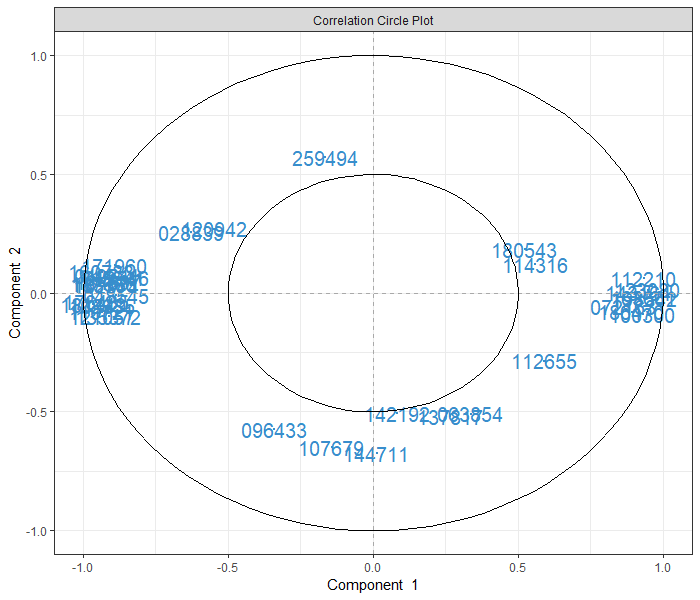FIGURE 5: Correlation circle plot representing the genes selected by MINT sPLS-DA performed on the stemcells gene expression data to examine the association of the genes selected on the first two components

Cluster Image Maps (CIMs) can be used to represent the gene expression levels of each sample for each loci. This is depicted in Figure 6. As was seen in Figure 1, the fibroblast cells (blue rows) cluster tightly together while the remaining two groups are less discernible from one another. The expression patterns within the fibroblast group are highly homogeneous across samples. This is not the case with the hiPSC and hESC groups.

cim(final.splsda.model, comp = 1, margins=c(10,5),
row.sideColors = color.mixo(as.numeric(Y)),
row.names = FALSE, title = "MINT sPLS-DA, component 1")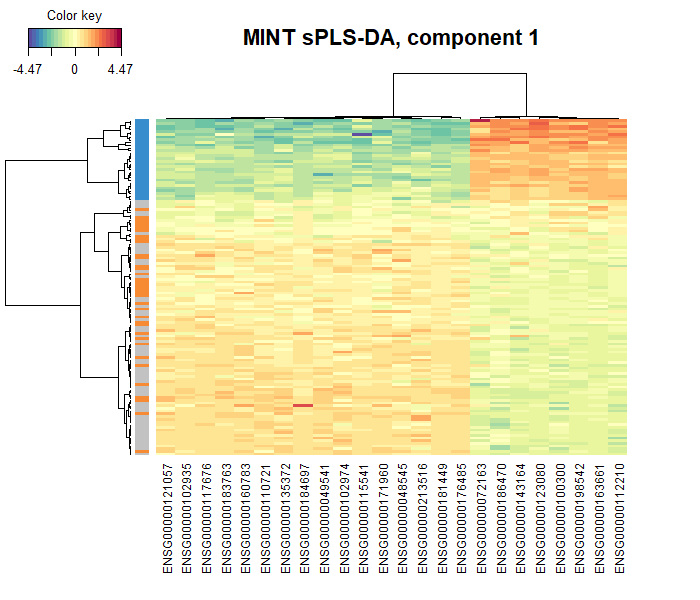FIGURE 6: Clustered Image Map of the genes selected by MINT sPLS-DA on the stemcells gene expression data for component 1 only. A hierarchical clustering based on the gene expression levels of the selected genes on component 1, with samples in rows coloured according to cell type

The last variable plot to be used is the Relevance network. This provides similar insights to the CIM above. Only the associations between the selected genes and the cell type (dummy encoded) are shown (Figure 7).

network(final.splsda.model, comp = 1,
color.node = c(color.mixo(1), color.mixo(2)),
shape.node = c("rectangle", "circle"))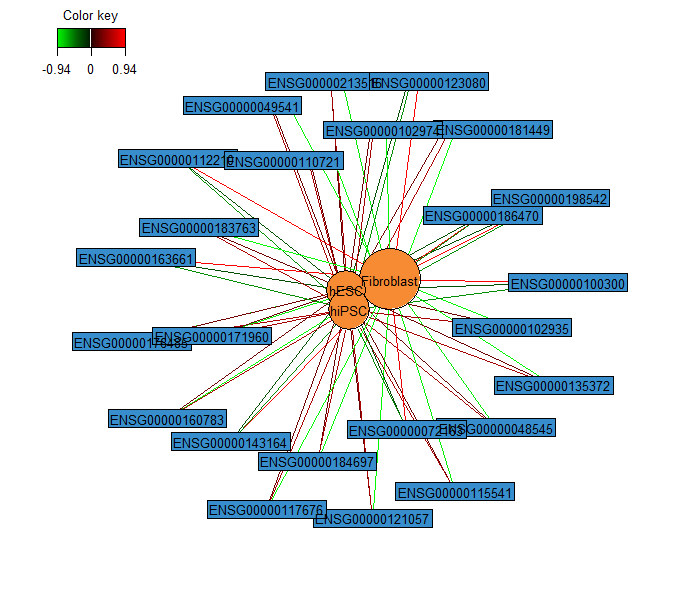FIGURE 7: Relevance network of the genes selected by MINT sPLS-DA performed on the stemcells gene expression data for component 1 only.

# Performance of the model

Use of the auroc() function will yield a visualisation of classification performance when undergoing the LOGOCV procedure from above. The interpretation of this output may not be particularly insightful in relation to the performance evaluation of our methods, but can complement the statistical analysis. For example, the model's classification of the fibroblast group had perfect specificity and sensitivity across the first component compared to the other two groups. (Figure 8).

auroc(final.splsda.model, roc.comp = 1, print = FALSE)
auroc(final.splsda.model, roc.comp = 2, print = FALSE)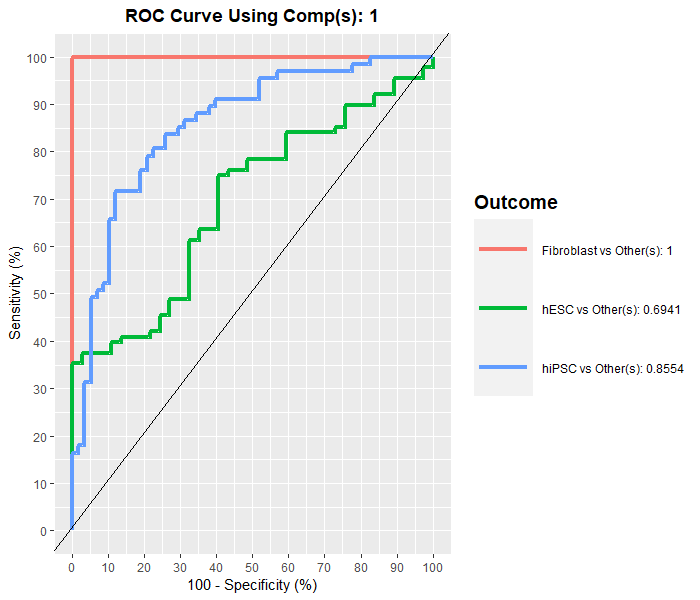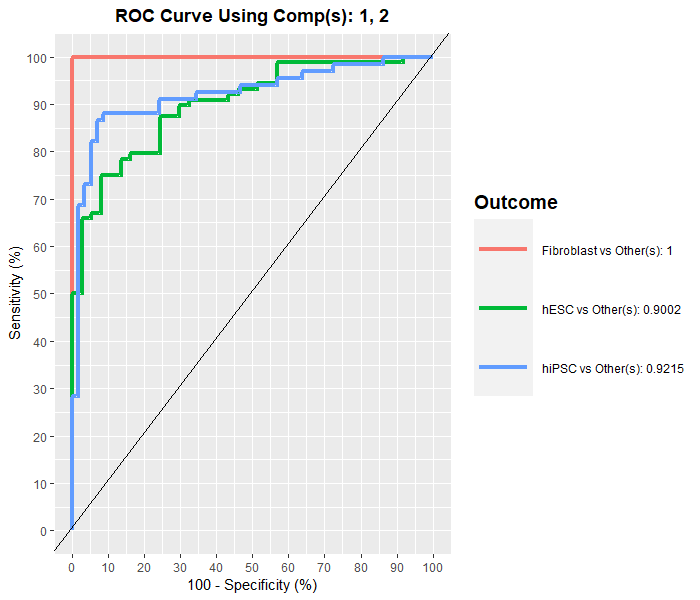FIGURE 8: ROC curve and AUC from the MINT sPLS-DA performed on the stemcells gene expression data for global component 1 (a) and component 2 (b), averaged across one-vs-all comparisons. Numerical outputs include the AUC and a Wilcoxon test p−value for each ‘one vs. other’ class comparison that are performed per component.

## Prediction on an external test set

To truly evaluate the performance of such a model in this context, the model should be trained and tested on separate datasets. Therefore, the third study will be sliced out to be used purely for testing. For simplicity, the tuned parameters (component and variable count) from above will be used rather than generating new ones.

From the below output, it can be seen that there were no fibroblast cells which were misclassified, while the hESC and hiPSC groups had 4 and 6 misclassifications respectively. The total prediction error rate was just short of 0.4 which is fairly high – the model could likely do with improvement (finer tuning and increased sample size).

# determine what rows correpsond to the third study
indiv.test <- which(study == "3")

# train a model on studies 1,2 and 4
perf.splsda.model <- mint.splsda(X = X[-c(indiv.test), ],
Y = Y[-c(indiv.test)],
study = droplevels(study[-c(indiv.test)]),
ncomp = optimal.ncomp,
keepX = optimal.keepX)

# make predictions of stem cell type of study 3 samples
predict.splsda <- predict(perf.splsda.model, newdata = X[indiv.test, ],
dist = "centroids.dist",
study.test = factor(study[indiv.test]))


The custom function PrintConfMat() does as the name would suggests: extracts the predicted labels and the true labels and forms them into a confusion matrix using a given number of components. YieldErrorRates() merely takes this confusion matrix and then returns the error rate and balanced error rate for a model using the inputted number of components.

PrintConfMat(1)

##            predicted.as.Fibroblast predicted.as.hESC predicted.as.hiPSC
## Fibroblast                       3                 0                  0
## hESC                             0                 4                  4
## hiPSC                            2                 2                  6


Interestingly, despite Figure 8 showing that the specificity and sensitivity of the model improving after the addition of the second component, the overall and balanced error rate actually increased. Looking at the confusion matrix below (for a model using the second component), the number of hiPSC samples that ended up being misclassified as hESC increased.

This is a very good example of why it is strongly advised that AUROC plots be used as purely complementary, and not for any kind of performance evaluation or model tuning.

YieldErrorRates(1)

## Metrics for model with 1 component(s):
## Error rate:  0.3809524
## Balanced error rate:  0.3

YieldErrorRates(2)

## Metrics for model with 2 component(s):
## Error rate:  0.4761905
## Balanced error rate:  0.3666667

PrintConfMat(2)

##            predicted.as.Fibroblast predicted.as.hESC predicted.as.hiPSC
## Fibroblast                       3                 0                  0
## hESC                             0                 4                  4
## hiPSC                            2                 4                  4